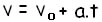Mechanics

# Braking and stopping

There is a difference between braking and stopping a car, a motorcycle or even a bicycle. This difference is defined by the Uniformly Varied Motion.

The difference between braking and stopping a piece of furniture has its definition based on the uniformly varied motion. This is due to the fact that the velocity undergoes equal variations in also equal intervals of time.

There is a condition for uniformly varied motion to occur: it is necessary that the average acceleration be equal to the instantaneous acceleration and its value must be different from zero.

We see in this definition that, in Uniformly Varied Motion (MUV), the velocity undergoes variations while the scalar acceleration is constant and non-zero.

When the brakes on a piece of furniture (car, motorcycle, bicycle, bus, etc.) are applied, it does not stop immediately, taking a while to come to a complete stop.

This difference between braking and stopping is easily observed on rainy days, because the water that is concentrated on the ground causes the car to “skid”, taking longer to stop.

There are conditions that we can analyze to physically understand this matter.

Reaction time – is the time it takes the driver to make the decision to brake the car or accelerate. It varies between 0.5 s and 1.0 s.

Braking Distance – is the distance traveled by the car at the moment of braking to the point where it actually stops. This distance can be longer or shorter; This depends on the condition of the tires, the asphalt and also the humidity.

Mobile Speed ​​– Another important factor is mobile speed. The higher its speed, the longer it will take to stop.

We can calculate the acceleration analysis introduced by the car’s brakes through the hourly speed function, represented by the mathematical equation:Where:
v = initial velocity
v0 = initial velocity
a= acceleration
t = time

There is also the possibility to calculate the total reaction distance of the conductor until the decision to stop the mobile. This calculation can be done using the equation:

d stop = d reaction + d braking

Where:
d stop = stopping distance
d reaction = reaction distance
d braking = braking distance

All these physical concepts serve to raise awareness of the hazards mainly encountered in traffic. It’s important to look both ways before crossing the street, think twice about running a red light and especially stay in front of heavier vehicles like buses and trucks. After all, between braking and stopping there is a difference!